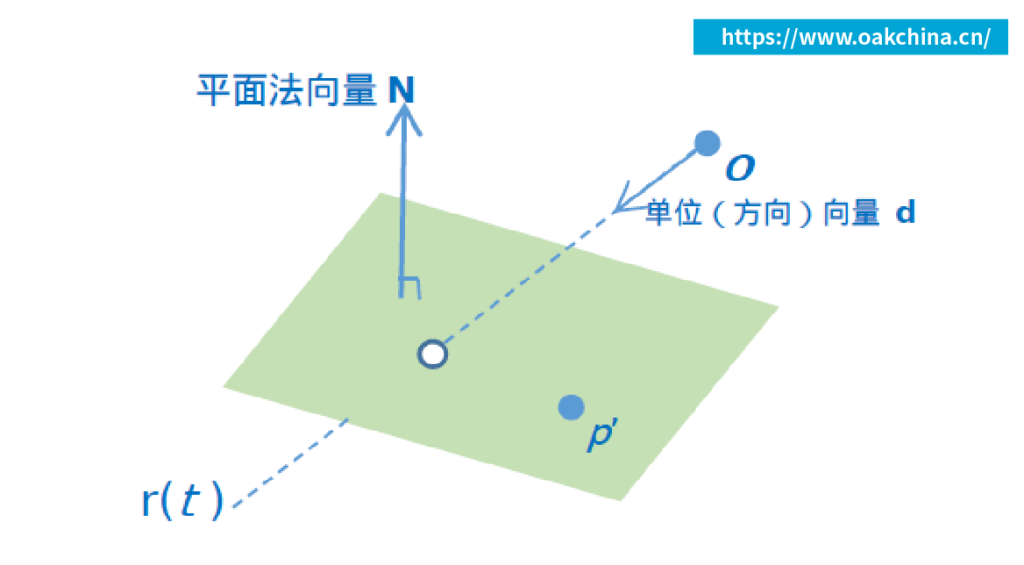+86-13951940532
contact@oakchina.cn

# 一文读懂射线与三角形相交算法Moller-Trumbore算法

## 一文读懂射线与三角形相交算法Moller-Trumbore算法## 1、三角形ABC 内的点P 的性质

```xvector = (B_x - A_x, C_x - A_x, A_x - P_x)
yvector = (B_y - A_y, C_y - A_y, A_y - P_y)
W = xvector 叉乘yvector ； 注意： 这里W 是向量[u, v, 1]，W 在x- y- z 坐标系中的坐标值为[u, v, 1]```

## 2、三角形Möller-Trumbore 算法（下文简称为M-T 算法）

Möller-Trumbore 算法的核心思想是一步到位的计算出光线是否与三角形相交，主要利用到的知识点是三角形的重心坐标。它是由Tomas Moller 和Ben Trumbore 于1997 年在一篇题名为“Fast, Minimum Storage Ray/Triangle Intersection”的论文中介绍。

### 2.2、M-T 算法

P = a A + u B + v C; 其中1 <= a 且u, v >= 0 且a + u + v = 1

o + t d = a A + u B + v C

o + t d = (1 – u – v ) A + u B + v C
=> o + t d = A – u A – v A + u B + v C
=> o + t d = A + (B – A)u + (C – A)v
=> o – A = -t d + (B-A) u + (C-A) v